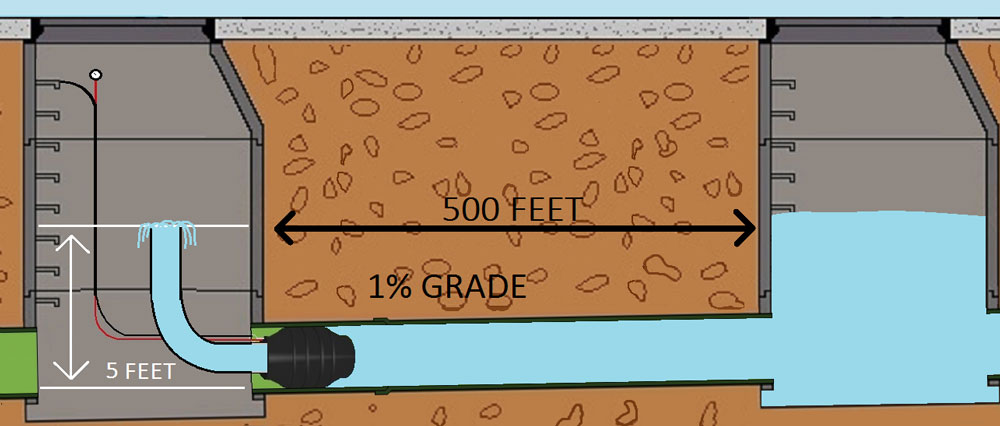# Tips from the Plug Peddler

### Pneumatic Plugs Essential Terminology.

Back Pressure: Back pressure is the amount of air or water that has built up behind (or in front of) a pipe plug.

Feet of Head or Head Pressure: The depth of the water from the centerline of the plug to the water’s surface.

Grade or Slope: The percentage the pipe is slanted downward to allow the affluent to flow. Example: If the pipe is on a 1 % grade that means the pipe gradually slants downward at a rate of 1 foot every 100 feet of pipe.

Pounds of Force: The amount of force being exerted on a pipe plug (back pressure). It is important to understand the pounds of force that a plug is holding back to help you understand the amount of damaged/danger that can be caused if a pipe plug fails or deflates. This also helps in developing or designing a proper blocking or bracing strategy.### Pneumatic Plugs Essential Calculations

Pounds of Force Formula:

3.14 x (radius of pipe²) = square inches or area of the pipe. Multiply this figure by PSI (back pressure) to calculate pounds of force.

Example: If a plug is installed in an 8-inch pipe and the plug is holding back 5 PSI of air pressure, how much force is the plug holding back?

1. Calculate the surface area of 8”-diameter pipe: 3.14 x 4(pipe radius)² = 50.24 square inches
2. Multiply the back pressure (5 PSI) x square inches: 5 x 50.24 = 251.20 pounds of force

Calculating feet of head using the slope or grade of the pipe:

To calculate the feet of head/head pressure that is backing up behind a pipe plug, you must know the grade or slope of the pipe and the distance the water is backing up behind the plug.

If a pipe is laid on a 1% grade and the water inside the pipe has backed up 500 feet behind the plug, then the plug will be holding back 5 feet of head.

Example: The number of feet the water is backed up in the pipe behind the plug equals 500 feet multiplied by the slope or grade. In this case equals 1% or .01 = (500 x .01 = 5 feet of head). Every additional 100 feet the water backs up would add one additional foot of head to the back pressure.

### Pneumatic Pipe Essential Conversions

General Conversion Table